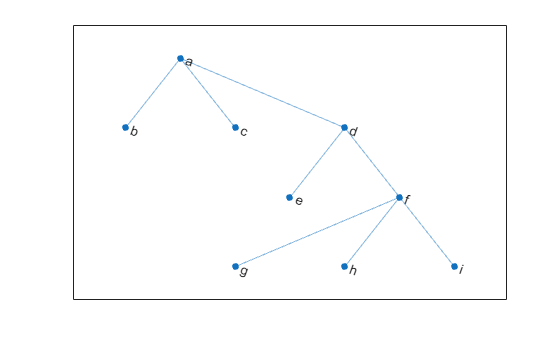# degree

Degree of graph nodes

## Syntax

``D = degree(G)``
``D = degree(G,nodeIDs)``

## Description

example

````D = degree(G)` returns the degree of each node in graph `G`. The degree is the number of edges connected to each node.```

example

````D = degree(G,nodeIDs)` returns the degree of the nodes specified by `nodeIDs`. ```

## Examples

collapse all

Create and plot a graph, and then use `degree` to find the degree of each node in the graph.

```s = [1 1 1 4 4 6 6 6]; t = [2 3 4 5 6 7 8 9]; G = graph(s,t); plot(G)````deg = degree(G)`
```deg = 9×1 3 1 1 3 1 4 1 1 1 ```

`deg(j)` indicates the degree of node `j`.

Create and plot a graph, and then find the degree of the first, third, and fifth nodes.

```s = {'a' 'a' 'a' 'd' 'd' 'f' 'f' 'f'}; t = {'b' 'c' 'd' 'e' 'f' 'g' 'h' 'i'}; G = graph(s,t); plot(G)``````nodeIDs = {'a' 'c' 'e'}'; deg = degree(G,nodeIDs)```
```deg = 3×1 3 1 1 ```

`deg(j)` indicates the degree of node `nodeIDs(j)`.

## Input Arguments

collapse all

Input graph, specified as a `graph` object. Use `graph` to create an undirected graph object.

Example: `G = graph(1,2)`

Node identifiers, specified as one or more node indices or node names.

This table shows the different ways to refer to one or more nodes either by their numeric node indices or by their node names.

FormSingle NodeMultiple Nodes
Node index

Scalar

Example: `1`

Vector

Example: `[1 2 3]`

Node name

Character vector

Example: `'A'`

Cell array of character vectors

Example: `{'A' 'B' 'C'}`

String scalar

Example: `"A"`

String array

Example: `["A" "B" "C"]`

Example: `D = degree(G,[3 4])`

Example: `D = degree(G,{'LAX','ALB'})`

## Output Arguments

collapse all

Degree of nodes, returned as a numeric array. `D` is a column vector unless you specify `nodeIDs`, in which case `D` has the same size as `nodeIDs`.

A node that is connected to itself by an edge (a self-loop) is listed as its own neighbor only once, but the self-loop adds 2 to the total degree of the node.